# Exercise E6.2 Lines and Angles NCERT Solutions Class 9

Go back to  'Lines and Angles'

## Chapter 6 Ex.6.2 Question 1

In the given figure, find the values of $$x$$ and $$y$$ and then show that $$AB ‖ CD$$.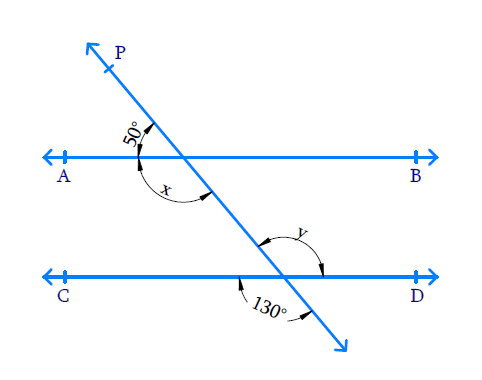### Solution

Reasoning:

• When two lines intersect, vertically opposite angles formed are equal.
• Also, when a ray intersects a line sum of adjacent angles formed is $$180^ {\circ}$$.
• If a transversal intersects two lines such that a pair of alternate angles is equal, then the two lines are parallel to each other.

Steps:

Line $$CD$$ is intersected with line $$P$$, hence the vertically opposite angles so formed are equal. $$y = 130^ {\circ}.$$

Similarly, line $$AB$$ is intersected by line $$P$$ hence the sum of adjacent angles formed is $$180^ {\circ}.$$

\begin{align} x + 50 ^ { \circ } & = 180 ^ { \circ } \\ x & = 180 ^ { \circ } - 50 ^ { \circ } \\ & = 130 ^ { \circ } \end{align}

We know that, if a transversal intersects two lines such that a pair of alternate interior angles is equal, then the two lines are parallel. Here we can see that the pair of alternate angles formed when lines $$AB$$ and $$CD$$ are intersected by transversal $$P$$ are equal. Therefore, $$x = y = 130^ {\circ}.$$ So we can say the two lines $$AB$$ and $$CD$$ are parallel. Hence $$AB ‖ CD$$ is proved.

## Chapter 6 Ex.6.2 Question 2

In the given figure, if $$AB\, ‖ \,CD\,,\; CD\, ‖ \,EF$$ and $$y:z = 3:7$$, find $$x$$.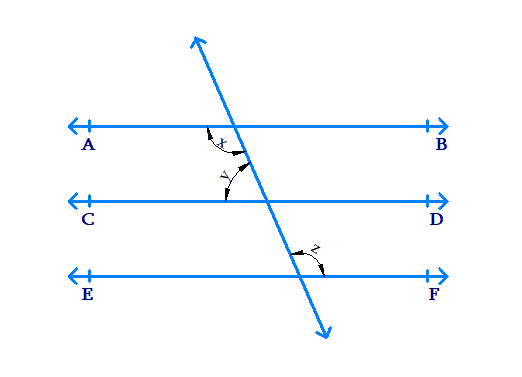### Solution

What is known?

$$AB ‖ CD$$

$$CD ‖ EF$$

$$y:z = 3:7$$ implies $$y = 3a$$ and $$z = 7a$$

What is unknown?

Value of $$x.$$

Reasoning:

• Lines which are parallel to the same line are parallel to each other.
• When two parallel lines are cut by a transversal, co-interior angles formed are supplementary.

Steps:

We know that, lines which are parallel to the same line are parallel to each other.

If $$AB ‖ CD$$, $$CD ‖ EF$$, we can say that $$AB ‖ EF$$.

Therefore, the angles $$x$$ and $$z$$ are alternate interior angles and hence are equal.

$x = z \qquad\ldots \ldots \ldots (1)$

$$AB$$ and $$CD$$ are parallel lines cut by transversal. So the co-interior angles formed are supplementary.

$x + y =180^ {\circ}$

$$\text{Since}\ x = z$$, we get $$y + z =180^ {\circ}$$

Given $$y = 3a, \;z = 7a$$

\begin{align} 3 a + 7 a &= 180 ^ { \circ } \\ 10 a &= 180 ^ { \circ } \\ a &= \frac { 180^ { \circ } } { 10 } \\ a &= 18 ^ { \circ } \end{align}

\begin{align} \therefore \qquad y & = 3 a \\ y &= 3 \times 18 ^ { \circ } \\ y &= 54 ^ { \circ } \end{align}

\begin{align} \therefore \qquad x + y &= 180 ^ { \circ } \\ x + 54 ^ { \circ } &= 180 ^ { \circ } \\ x &= (180 ^ { \circ } - 54 ^ { \circ }) \\ x &= 126 ^ { \circ } \end{align}

## Chapter 6 Ex.6.2 Question 3

In the given figure, if $$AB \,‖\, CD$$, $$EF \,\bot \,CD$$ and $$\angle GED = 126^ {\circ},$$ find $$\angle AGE, \; \angle GEF$$ and $$\angle FGE.$$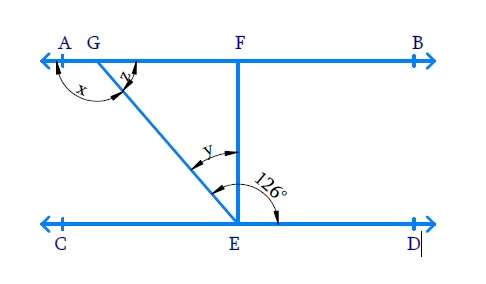### Solution

What is known?

$$AB ‖ CD$$, $$\text{EF} \bot \text{CD}$$ and $$\angle GED = 126^ {\circ},$$

What is unknown?

$$\angle AGE =?,\;\; \angle GEF=?$$ and $$\angle FGE =?$$

Reasoning:

• When two lines intersect, adjacent angles formed are supplementary.
• When two parallel lines are cut by a transversal, alternate interior angles formed are equal.

Steps:

Let $$\angle AGE = x,\;\; \angle GED= y$$ and $$\angle FGE = z$$.

From the figure, we can see that,

\begin{align} \angle GED& = \angle GEF + \angle FED \\ y & = (126 ^ { \circ } - 90 ^ { \circ }) \\ \angle GEF & = y = 36 ^ { \circ } \end{align}

$$AB$$ and $$CD$$ are parallel lines cut by a transversal, the pair of alternative angles formed are equal.

\begin{align} \angle AGE &= \angle GED \\ \angle AGE &= x = 126 ^ { \circ } \end{align}

Line $$AB$$ is intersected by line $$GE$$ hence adjacent angles formed are supplementary.

\begin{align} x + z &= 180 ^ { \circ } \\ 126 ^ { \circ } + z &= 180 ^ { \circ } \\ z &= 180 ^ { \circ } - 126 ^ {\circ } \\ \quad &= 54 ^ {\circ} \\ \angle FGE = z &= 54 ^ { \circ } \end{align}

## Chapter 6 Ex.6.2 Question 4

In the given figure, if $$PQ || ST$$, $$\angle PQR = 110^ \circ$$ and $$\angle RST = 130^ {\circ}$$, find $$\angle QRS$$.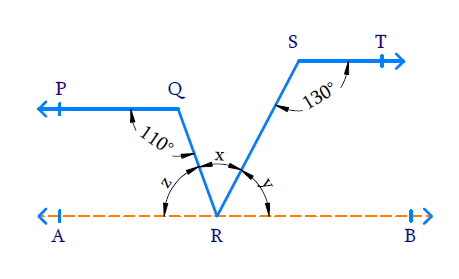### Solution

What is known?

$$PQ \| ST, \angle PQR = 110 ^ { \circ }$$ and $$\angle RST = 130 ^ { \circ }$$

What is unknown?

$$\angle QRS =?$$

Reasoning:

• Lines which are parallel to the same line are parallel to each other.
• When two parallel lines are cut a transversal, co-interior angles formed are supplementary.

Steps:

Draw a line $$AB$$ parallel to $$ST$$ through point $$R$$. Since $$AB\|ST$$ and $$PQ\|ST$$. So, $$AB\|PQ$$.

Let $$\angle SRQ = x,\;\; \angle SRB = y$$ and $$\angle QRA = z$$

Lines $$ST$$ and $$AB$$ are parallel with transversal $$SR$$ intersecting. Therefore, the co-interior angles are supplementary.

\begin{align} \angle RST + \angle SRB & = 180 ^ { \circ } \\ 130 ^ { \circ } + y & = 180 ^ { \circ } \\ y & = (180 ^ { \circ } - 130 ^ { \circ }) \\ & = 50 ^ { \circ } \\ \angle SRB = y & = 50 ^ { \circ } \end{align}

Similarly, lines $$PQ$$ and $$AB$$ are parallel with transversal $$QR$$ intersecting. Therefore, the co-interior angles are supplementary.

\begin{align} \angle PQR + \angle QRA & = 180 ^ { \circ } \\ 110 ^ { \circ } + z & = 180 ^ { \circ } \\ z & = (180 ^ { \circ } - 110 ^ { \circ }) \\ & = 70 ^ { \circ } \\ \angle QRA = z & = 70 ^ { \circ } \end{align}

$$AB$$ is a line, $$RQ$$ and $$RS$$ are rays on $$AB$$. Hence,

\begin{align} \angle QRA + \angle QRS + &\angle SRB = 180 ^ { \circ } \\ 70 ^ { \circ } + x + 50 ^ { \circ } & = 180 ^ { \circ } \\ 120 ^ { \circ } + x & = 180 ^ { \circ } \\ x & = (180 ^ { \circ } - 120 ^ { \circ }) \\ x & = 60 ^ { \circ } \\ \angle QRS = x & = 60 ^ { \circ } \end{align}

## Chapter 6 Ex.6.2 Question 5

In the given figure, if $$AB \| CD,\;\; \angle APQ = 50 ^ { \circ }$$ and $$\angle PRD = 127 ^ { \circ }$$, find $$x$$ and $$y$$.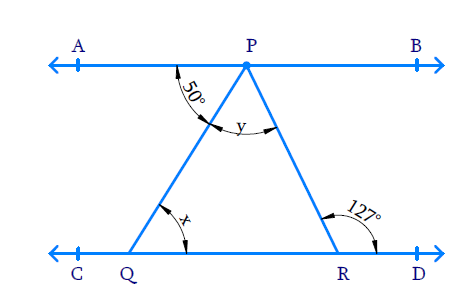### Solution

What is known?

$$AB \| CD,\;\; \angle APQ = 50 ^ { \circ }$$ and $$\angle PRD = 127 ^ { \circ }$$

What is unknown?

$$x =?$$ and $$y = ?$$

Reasoning:

• When a ray intersects a line, sum of adjacent angles formed is $$180^ {\circ}$$.
• When two parallel lines are cut by a transversal, alternate interior angles formed are equal.

Steps:

$$AB$$ and $$CD$$ are parallel lines cut by transversal $$PQ$$ hence the alternate interior angles formed are equal.

$$\angle APQ = \angle PQR$$ and hence $$x = 50^ {\circ}$$.

Similarly, $$AB$$ and $$CD$$ are parallel lines cut by transversal $$PR$$ hence the alternate angles formed are equal.

\begin{align} \angle APR+ \angle PRD &= 127 ^ { 0 } \\ \angle APQ + \angle QPR &= \angle PRD \\&= 127 ^ { \circ } \\ 50 ^ { \circ } + y &= 127 ^ { 0 } \\ y &= (127 ^ { 0 } - 50 ^ { \circ }) \\ y &= 77 ^ { \circ } \end{align}

## Chapter 6 Ex.6.2 Question 6

In the given figure, $$PQ$$ and $$RS$$ are two mirrors placed parallel to each other. An incident ray $$AB$$ strikes the mirror $$PQ$$ at $$B$$, the reflected ray moves along the path $$BC$$ and strikes the mirror $$RS$$ at $$C$$ and again reflects back along $$CD$$.

Prove that $$AB \|CD$$.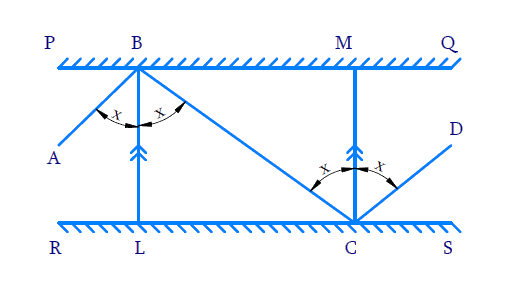### Solution

What is known?

$$PQ \| RS$$

What is unknown?

To prove: $$AB \| CD$$

Reasoning:

When two parallel lines are cut by a transversal, alternate angles formed are equal.

In optics the angle of incidence (the angle which an incident ray makes with a perpendicular to the surface at the point of incidence) and the angle of reflection (the angle formed by the reflected ray with a perpendicular to the surface at the point of incidence) are equal.

Steps:

Draw perpendicular lines $$BL$$ and $$CM$$ at the point of incident on both mirrors since $$PQ$$ and $$RS$$ parallel to each other, perpendiculars drawn are parallel $$BL \| CM$$. Since $$BC$$ is a transversal to lines $$BL$$ and $$CM$$, alternate angles are equal so we get

$\angle LBC = \angle BCM = x \;(\text{say})\; \ldots ( 1 )$

By laws of reflection, at the first point of incidence $$B$$, we get:

\begin{align} \angle ABL & = \angle LBC = x \\ \therefore \angle ABC& = \angle ABL+ \angle LBC \\ & = x + x \\ \therefore \angle ABC & = 2 x \ldots \ldots \ldots ( 2 ) \end{align}

By laws of reflection, at the first point of incidence $$C$$, we get:

\begin{align} \angle MCD & = \angle BCM = x \\ \therefore \angle BCD& = \angle BCM + \angle MCD \\ & = x + x \\ \angle BCD &= 2 x \ldots \ldots ( 3 ) \end{align}

From equations ($$2$$) and ($$3$$), we get $$\angle ABC = \angle BCD$$.

We know that, if a transversal intersects two lines such that a pair of alternate interior angles is equal, then the two lines are parallel. As Alternate angles are equal we can say $$AB \| CD$$.

Related Sections
Related Sections
Instant doubt clearing with Cuemath Advanced Math Program